# Equilateral triangle facts for kids

Kids Encyclopedia Facts

In geometry, an equilateral triangle is a triangle where all three sides are the same length and all three angles are also the same and are each 60°. If an altitude is drawn, it bisects the side to which it is drawn, in turn leaving two separate 30-60-90 triangles

## Principal properties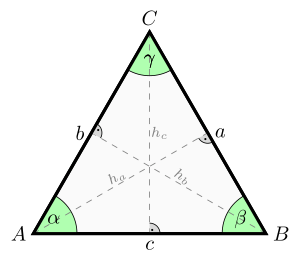An equilateral triangle. It has equal sides (a=b=c), equal angles (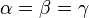$\alpha = \beta =\gamma$), and equal altitudes (ha=hb=hc).

Denoting the common length of the sides of the equilateral triangle as a, we can determine using the Pythagorean theorem that:

• The area is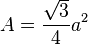$A=\frac{\sqrt{3}}{4} a^2$
• The perimeter is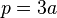$p=3a\,\!$
• The radius of the circumscribed circle is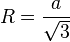$R = \frac{a}{\sqrt{3}}$
• The radius of the inscribed circle is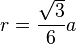$r=\frac{\sqrt{3}}{6} a$ or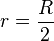$r=\frac{R}{2}$
• The geometric center of the triangle is the center of the circumscribed and inscribed circles
• And the altitude (height) from any side is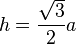$h=\frac{\sqrt{3}}{2} a$.

Denoting the radius of the circumscribed circle as R, we can determine using trigonometry that:

• The area of the triangle is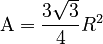$\mathrm{A}=\frac{3\sqrt{3}}{4}R^2$

Many of these quantities have simple relationships to the altitude ("h") of each vertex from the opposite side:

• The area is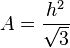$A=\frac{h^2}{\sqrt{3}}$
• The height of the center from each side, or apothem, is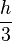$\frac{h}{3}$
• The radius of the circle circumscribing the three vertices is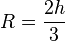$R=\frac{2h}{3}$
• The radius of the inscribed circle is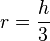$r=\frac{h}{3}$

In an equilateral triangle, the altitudes, the angle bisectors, the perpendicular bisectors and the medians to each side coincide.

## Geometric construction

An equilateral triangle is easily constructed using a compass and straightedge, as 3 is a Fermat prime. Draw a straight line, and place the point of the compass on one end of the line, and swing an arc from that point to the other point of the line segment. Repeat with the other side of the line. Finally, connect the point where the two arcs intersect with each end of the line segment

An alternative method is to draw a circle with radius r, place the point of the compass on the circle and draw another circle with the same radius. The two circles will intersect in two points. An equilateral triangle can be constructed by taking the two centers of the circles and either of the points of intersection.

In both methods a by-product is the formation of vesica piscis.

The proof that the resulting figure is an equilateral triangle is the first proposition in Book I of Euclid's Elements.

## Derivation of area formula

The area formula$A = \frac{\sqrt{3}}{4}a^2$ in terms of side length a can be derived directly using the Pythagorean theorem or using trigonometry.Equilateral triangle Facts for Kids. Kiddle Encyclopedia.